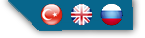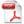ISSN: 2149-3561 Yeni Üye | Unuttum HAKEM & EDİTÖR GİRİŞİ
Avrasya Ekonometri ï¿½statistik ve Ampirik Ekonomi Dergisi Yıl:2016  Sayı: 5  Alan: ï¿½statistik-Sayï¿½sal Yï¿½ntemler-EkonometriKadir GÜÇ, Emel BAŞAR
Optimal Ölçeklemeye Dayalı Kategorik Regresyon Analizi ve Bir Uygulama

Bağımlı değişkeni kategorik olan modelleri açıklama amacı taşıyan lojistik regresyon analizi, sosyal bilim çalışmalarında sıklıkla kullanılmaktadır. Alternatif tekniklere göre varsayımlarının azlığı ve çıktılarının kolay yorumlanabilmesi, lojistik regresyon analizini cazip bir hale getirmektedir. Benzer şekilde optimal ölçekleme olarak bilinen teknikler de, kategorik değişkenlerin analizinde kullanılan bir diğer teknikler kümesi olarak karşımıza çıkmaktadır. Optimal ölçekleme tekniklerinden olan ve tıpkı lojistik regresyon gibi bağımlı değişkeni kategorik modelleri açıklamada kullanılan bir diğer teknik ise kategorik regresyon analizidir. Kategorik regresyon analizi, tıpkı lojistik regresyon gibi az sayıda varsayıma dayanmakta ve son derece etkin çözümler üretmektedir. Bu çalışmada optimal ölçeklemeye dayalı kategorik regresyon analizinin yapısı ve özellikleri incelenmeye çalışılmıştır. Bu amaçla öncelikle optimal ölçekleme teknikleri kısaca anlatılmış, daha sonra kategorik regresyon analizinin yapısına dair bilgiler aktarılmıştır. Çalışmanın uygulama bölümünde Bilgiç ve Akyürek (2009) tarafından hazırlanan Güneydoğunun Sosyolojik Analizi isimli çalışmadaki veriden yararlanılarak ülkemizde medyaya duyulan güven ile çeşitli demografik değişkenler arasındaki ilişkiler kategorik regresyon analizi ile incelenmiştir. Böylelikle kategorik regresyonun, lojistik regresyona alternatif bir teknik olabileceği ve kategorik değişkenlerin yer aldığı modellerde, kategorik regresyon ile çözümlemelerin yapılabileceği sonucuna varılmıştır.

Anahtar Kelimeler: Kategorik regresyon, optimal ï¿½lï¿½ekleme, kategorik veri

Categorical Regression Based on Optimal Scaling and An Application

Logistic regression analysis which aims to explain the models whose dependent variable is categorical is frequently used in social science studies. In comparison with alternative techniques, exiguity of assumptions and easy interpretation of outputs make logistic regression analysis attractive. Similarly, the techniques which are known optimal scaling are used in the analysis of categorical variables. Just like logistic regression, another optimal scaling technique that aims to explain the models whose dependent variable is categorical is categorical regression analysis. Also, similar to logistic regression analysis, categorical regression has few assumptions and produce highly effective solutions. In this study the structure and properties of categorical regression analysis that based on optimal scaling are investigated. For this purpose, optimal scaling techniques are explained briefly, then information about the structure of the categorical regression analysis is described. In the application part of the study, by benefiting from the study named ï¿½Sociological Analysis of Southeast Problemï¿½ by Bilgiï¿½ and Akyï¿½rek (2009), the relationships between the confidence level in media and various demographic variables will be investigated using categorical regression analysis. Thus, it is deduced that the categorical regression could be an alternative technique to logistic regression and models involving categorical variables were concluded with the categorical regression analysis can be made.

Keywords: Categorical regression (CATREG), optimal scaling, categorical dataDetayİÇERİK